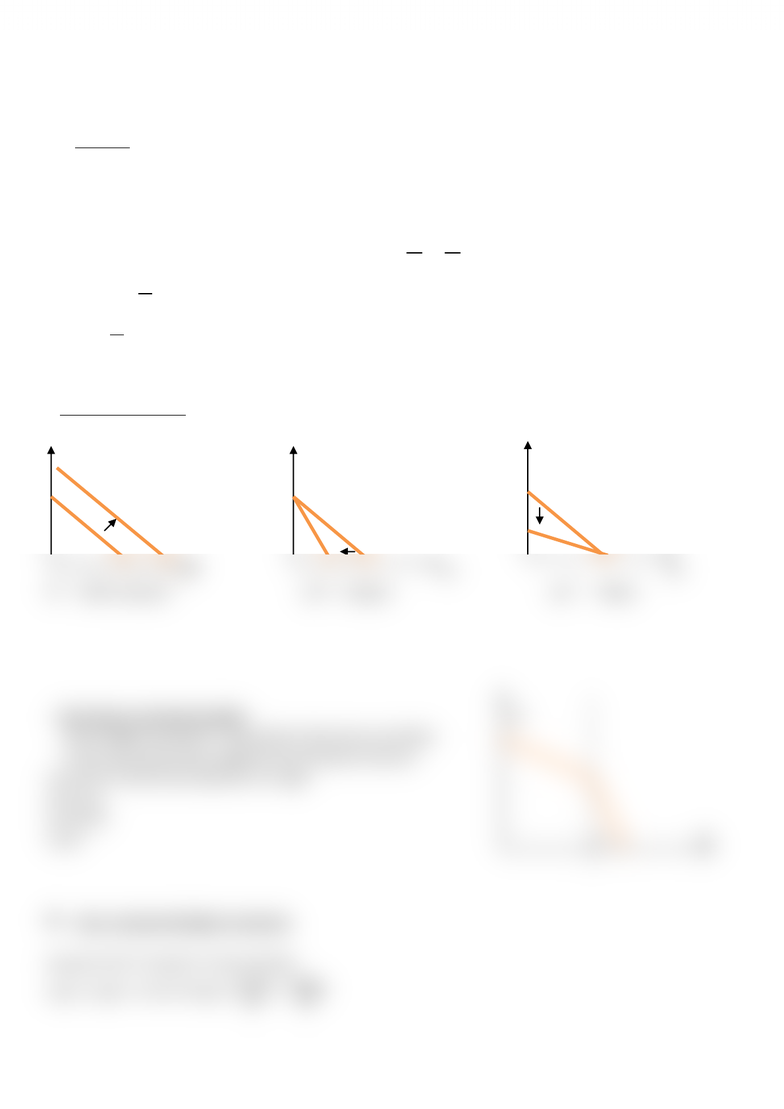Class Notes (1,100,000)
CA (650,000)
UTSG (50,000)
ECO (2,000)
Lecture

# Topic 3 - Budget Constraints

Department
Economics
Course Code
ECO206Y1
Professor
Loren Brandt

This preview shows half of the first page. to view the full 2 pages of the document.Topic 3 - Budget Constraints
(Lecture 2 Sept 21st)
Intuition:
1. Goods: x1, x2;
2. Price: p1, p2
3. E: total expenditure/budget the individual has;
Assume that all of E is spent, E = p1x1 + p2x2 


Y-intercepts:
 = maximum units of x2 that can be consumed;
Slope: 
 = OC = Units of x2 that have to be reduced to consume one more unit of x1;
-- Change in Variables:
x2 x2 x2
x1 x1 x1
E shifts outward p1 steeper p2 flatter
-- Non-linear Constraint budget x2
Liner budget constraint = assumption that prices are fixed;
In the real world, there might be non-linearity of prices
e.g. price of electricity depends on usage
x1<a, p1;
x1>a,2p1;
x2,p2 a x1
Inter- temporal Budget Constraint
Assume Y1,Y2 “income” in two periods;
x1p1 + x2p2 = Y1+Y2 x1 = 
 
